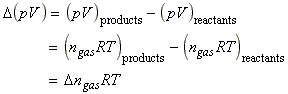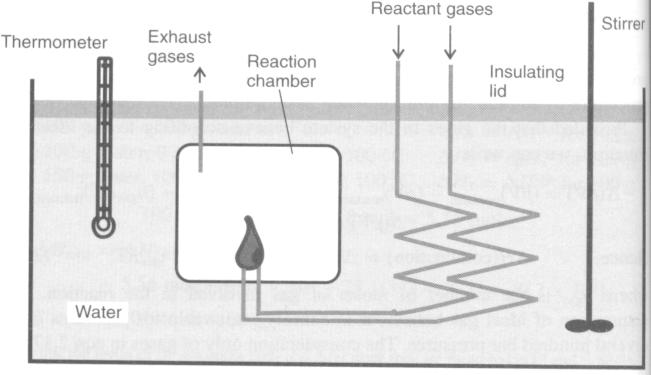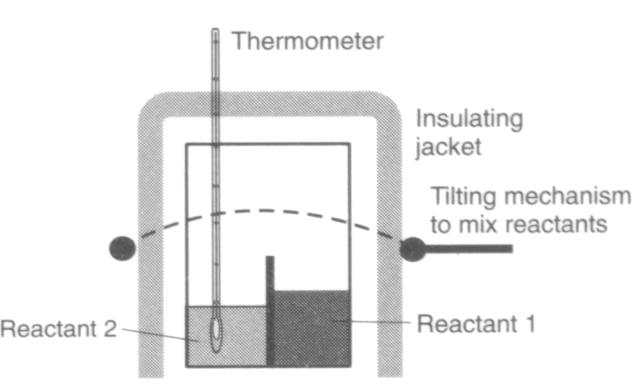Back to Table of Contents [TOP] Measurement of DH Enthalpy changes are measured directly in a calorimeter This involves measuring the temperature change when known amounts of compounds react usually in an insulated container. In this section we shall discuss the three main types of calorimeters, namely:             (1) The bomb calorimeter (Fig. 1)            (2) The flame calorimeter (Fig. 2)            (3) Solution calorimeter. (Fig. 3) In addition to direct calorimetry, other methods for measuring enthalpy changes in redox reactions are based on the variation of the electrochemical properties of the reaction These shall be considered in CH308. [ TOP ] The bomb calorimeter The first type of calorimeter uses an indirect method for calculating enthalpies of combustion, DH(combustion) from DU(combustion).  The change in internal energy during a reaction is simply the heat change during the reaction if it is conducted at constant volume. DU during a combustion reaction can be measured using a bomb calorimeter, shown schematically in Fig. 1. The reaction chamber or bomb which contains the substance under study is constructed from thick (c. 1 cm) stainless steel, ensuring that the system volume does not change. After pressurizing with oxygen gas, the reaction can be started by electrically heating a piece of wire in contact with the reactants. The heat given out on combustion warms up both the metal of the calorimeter and the water that surrounds it. The temperature is measured precisely to +/-0.0001K with an accurate thermometer. If we could know the effective heat capacity, Ccalorimeter, of the calorimeter, we could calculate the internal energy change by:(Eqn. 1) where the negative sign arises from our sign convention, since the heat is given out from the system. In theory, the Ccalorimeter can be measured from " Ccalorimeter = mwatercwater + msteelcsteel " but in practice, Ccalorimeter is determined by a calibration. The temperature rise when a known mass of a compound (usually naphthalene or benzoic acid) with accurately known DU(combustion) undergoes reaction is measured and fitted to eqn. 1 to calculate Ccalorimeter . Alternatively, an electrical calibration can be performed. If a current I passes through the wire at a voltage V for time t,the energy delivered is given by (E = VIt). Measurement of the temperature increase caused by this energy applied to a wire inside the bomb allows Ccalorimeter to be calculated.  The value of DU(combustion) can be readily converted to DH(combustion) using the definition below:  DH (combustion) = DU (combustion) + D (pV)(combustion) Since the volume is constant, the last term suggests that the pressure in the calorimeter must be measured. However, we can simplify the procedure by introducing the following assumptions: (1) Provided that the gases in the system behave according to the ideal gas equation, we can write:(2) Because of the very big differences between the molar volume of gases and liquids/solids, we do not need to consider the effect of solids/liquids - the differences between the internal energy and enthalpy are relatively small in these cases. NOTE: This apparatus is not suitable for measuring the properties of gas reactions. These are usually performed in a flame calorimeter, see below. [ TOP ] Flame calorimeter.  The flame calorimeter (see fig. 2) is used to measure the properties of gas reactions (see fig. 2). The gases concerned are fed at a known, constant rate to a jet at which the reaction occurs. The reaction chamber and gas pipes are contained in a thermostatically controlled water bath to ensure constant temperature. The reaction is then started and the temperature rise measured after a known amount of gas has been fed into the reaction. Calibration of the calorimeter, either with a standard reaction or by electrical means (similar to that described for the bomb calorimeter), allows calculation of DH(reaction) since the reaction is conducted at constant pressure. Example 2.9 illustrates the use of this type of apparatus for measuring an enthalpy of combustion where one of the gases used is oxygen. [ TOP ] Solution calorimeter Solution calorimeters (see fig. 3) are commonly used for measuring heat changes during reactions that occur in solution. It allows two (or more) reactants to be placed in an insulated, thermostatted chamber until thermal equilibrium is reached. The reactants can then be rapidly mixed to start the reaction, either by tilting the reaction vessel, or, in more sophisticated versions, using a magnet to break a glass seal between the chambers. Calibration of this type of apparatus is often carried out using a neutralization reaction between a strong acid and a strong base. (The enthalpy change for this reaction is -56.9 kJ mol-1.)   [ TOP ] Figures:Fig. 1: The Bomb Calorimeter [ TOP ]Fig. 2: The Flame Calorimeter [ TOP ]Fig. 3: The Solution Calorimeter [ TOP ]CH237 - Chemical Thermodynamics and Kinetics

 BACK TO TABLE OF CONTENTS   Measurment of DH Enthalpy changes are measured directly in a calorimeter This involves measuring the temperature change when known amounts of compounds react usually in an insulated container. In this section we shall discuss the three main types of calorimeters, namely:             (1) The bomb calorimeter (Fig. 1)            (2) The flame calorimeter (Fig. 2)            (3) Solution calorimeter. (Fig. 3) In addition to direct calorimetry, other methods for measuring enthalpy changes in redox reactions are based on the variation of the electrochemical properties of the reaction These shall be considered in CH308. [ TOP ] The bomb calorimeter The first type of calorimeter uses an indirect method for calculating enthalpies of combustion, DH(combustion) from DU(combustion).  The change in internal energy during a reaction is simply the heat change during the reaction if it is conducted at constant volume. DU during a combustion reaction can be measured using a bomb calorimeter, shown schematically in Fig. 1. The reaction chamber or bomb which contains the substance under study is constructed from thick (c. 1 cm) stainless steel, ensuring that the system volume does not change. After pressurizing with oxygen gas, the reaction can be started by electrically heating a piece of wire in contact with the reactants. The heat given out on combustion warms up both the metal of the calorimeter and the water that surrounds it. The temperature is measured precisely to +/-0.0001K with an accurate thermometer. If we could know the effective heat capacity, Ccalorimeter, of the calorimeter, we could calculate the internal energy change by:(Eqn. 1) where the negative sign arises from our sign convention, since the heat is given out from the system. In theory, the Ccalorimeter can be measured from " Ccalorimeter = mwatercwater + msteelcsteel " but in practice, Ccalorimeter is determined by a calibration. The temperature rise when a known mass of a compound (usually naphthalene or benzoic acid) with accurately known DU(combustion) undergoes reaction is measured and fitted to eqn. 1 to calculate Ccalorimeter . Alternatively, an electrical calibration can be performed. If a current I passes through the wire at a voltage V for time t,the energy delivered is given by (E = VIt). Measurement of the temperature increase caused by this energy applied to a wire inside the bomb allows Ccalorimeter to be calculated.  The value of DU(combustion) can be readily converted to DH(combustion) using the definition below:  DH (combustion) = DU (combustion) + D (pV)(combustion) Since the volume is constant, the last term suggests that the pressure in the calorimeter must be measured. However, we can simplify the procedure by introducing the following assumptions: (1) Provided that the gases in the system behave according to the ideal gas equation, we can write:(2) Because of the very big differences between the molar volume of gases and liquids/solids, we do not need to consider the effect of solids/liquids - the differences between the internal energy and enthalpy are relatively small in these cases. NOTE: This apparatus is not suitable for measuring the properties of gas reactions. These are usually performed in a flame calorimeter, see below. [ TOP ] Flame calorimeter.  The flame calorimeter (see fig. 2) is used to measure the properties of gas reactions (see fig. 2). The gases concerned are fed at a known, constant rate to a jet at which the reaction occurs. The reaction chamber and gas pipes are contained in a thermostatically controlled water bath to ensure constant temperature. The reaction is then started and the temperature rise measured after a known amount of gas has been fed into the reaction. Calibration of the calorimeter, either with a standard reaction or by electrical means (similar to that described for the bomb calorimeter), allows calculation of DH(reaction) since the reaction is conducted at constant pressure. Example 2.9 illustrates the use of this type of apparatus for measuring an enthalpy of combustion where one of the gases used is oxygen. [ TOP ] Solution calorimeter Solution calorimeters (see fig. 3) are commonly used for measuring heat changes during reactions that occur in solution. It allows two (or more) reactants to be placed in an insulated, thermostatted chamber until thermal equilibrium is reached. The reactants can then be rapidly mixed to start the reaction, either by tilting the reaction vessel, or, in more sophisticated versions, using a magnet to break a glass seal between the chambers. Calibration of this type of apparatus is often carried out using a neutralization reaction between a strong acid and a strong base. (The enthalpy change for this reaction is -56.9 kJ mol-1.)   [ TOP ] Figures:Fig. 1: The Bomb Calorimeter [ TOP ]Fig. 2: The Flame Calorimeter [ TOP ]Fig. 3: The Solution Calorimeter [ TOP ] BACK TO TABLE OF CONTENTS

[ UNIVERSITY OF MALTA | FACULTY OF SCIENCE | DEPARTMENT OF CHEMISTRY ]

 E-mail me at jgri1@um.edu.mt http://staff.um.edu.mt/jgri1